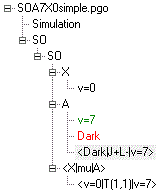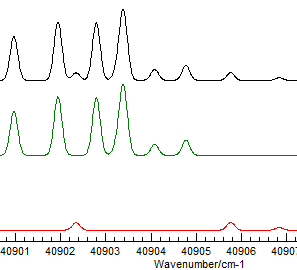Molecule Types Linear Molecules Samples

# A Perturbation in SO

This file reproduces the isolated perturbation required to simulate v' = 7 of the A 3Π state in SO, as described in "The A 3Π state of SO", J M F Elks and C M Western, J. Chem. Phys. 110, 7699 (1999). See equation 6, and figures 4 and 5. To simulate the perturbation, an extra state, here labelled "Dark", is added to the upper manifold (for the A state), and a single perturbation term (Luncouple, equivalent to J+L-+J-L+) is added linking this state with v' = 7 of the A state. Two data files are provided:• SOA7X0simple.pgo - a slightly simplified file, with just the perturbation above included. The diagram to the left shows the structure of the file.
• SOA7X0full.pgo - the file required to reproduce the results in the paper, which required the some adjustments to the B values for the Ω = 0 and 2 components.
The plot below shows a fragment of the simulation, with the upper (black) trace the overall simulation, the middle (green) trace being transitions from the A state and the lower (red) trace the transitions from the perturbing state.